# Natural numbers - 7th grade (12y) - math problems

#### Number of problems found: 241

• Lcm = 22 + gcdThe least common multiple of two numbers is 22 more than their greatest common divisor. Find these numbers.
• MartinaMartina is solving the equation 4x - 11 = 2x + 391. Here are the first steps of her solution. 4x - 11 = 2x + 391 2x - 11 = 391 2x = 402 What did Martina do to get 2x - 11 = 391?
• MarshallMarshall earns a salary of 36,000 in each year and he receives a \$4000 raise Jim and the salary of 51,000 each year and he receives a 1500 rays how many years will it take before Marshall and Jim are in the same salary
• Phone number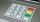Ivan's phone number ends with a four-digit number: When we subtract the first from the fourth digit of this four-digit number, we get the same number as when we subtract the second from the third digit. If we write the four-digit number from the back and
• How many 17How many fifths are there in four wholes? How many 1/5 are there in 4?
• Athletics teamAll athletes in the athletics team from Ostrava can start in four, five, six and seven stages, and no one will be present. How many average athletes are in one athletic group if there are a total of twelve groups in the section? Consider the smallest numb
• Three integersThree consecutive integers add up to 51. what are these integers?
• A class IV.CIn a class 2/5 were boys. 30 were girls. How many more girls than boys are there?
• Six times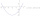6 times a number has 2 subtracted from it. The result it thrilled . the answer is the same if you add 6 to twice the number and multiply the result by 2
• English and math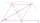1/5 of a class study English, and 3/5 study mathematics. If the class consist of 80 students, how many students study English and mathematics in the class?
• SixteenSixteen minus the quotient of 12 and what number is thirteen?
• The largest divisorThe largest number which divides 60 and 75 leaving remainders 8 and 10 respectively is:
• What are 3What are the two digits which when inserted in the blank spaces will make 234 _ _ divisible by 8?
• Four multiplesFour multiples of 6 he writes the following 12  24  56  72, which is correct?
• A number 5A number is divisible by 24, 25, and 120 if it is increased by 20. Find the number.
• The cost 2The cost of 5 apples is \$3.45  and 5 oranges is \$1.23. If Rachel buys one apple and one orange, then how much must she pay?
• The buildingThere are 45 people living in building, out of these 3/5 are men,2/5 are women . find the number of men and women in the building.
• Players - baseball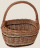There are 20 players on each of two baseball teams. If 2/5 of the players on team 1 miss practice and 1/4 of the players on team two miss practice, how many more players from team 1 missed practice then team 2?
• What fraction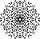What fraction of number 1 to 30 is prime?
• EmberEmber spent \$144 on her birthday party for 18 guests. If the cost is at the same rate, how many guests will there if she spends \$176?

Do you have an exciting math question or word problem that you can't solve? Ask a question or post a math problem, and we can try to solve it.

We will send a solution to your e-mail address. Solved examples are also published here. Please enter the e-mail correctly and check whether you don't have a full mailbox.

Natural numbers - math problems. Examples for 7th grade (seventh).